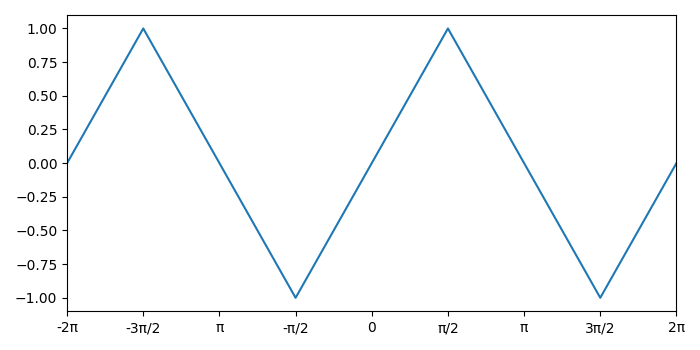# How to set axis ticks in multiples of pi in Python Matplotlib?

To set axis ticks in multiples of pi in Python, we take following steps −

• Initialize a pi variable, create theta and y data points using numpy.

• Plot theta and y using plot() method.

• Get or set the current tick locations and labels of the X-axis using xticks() method.

• Convenience method to set or retrieve autoscaling margins using margins() method.

• To display the figure, use show() method.

## Example

import numpy as np
from matplotlib import pyplot as plt
plt.rcParams["figure.figsize"] = [7.00, 3.50]
plt.rcParams["figure.autolayout"] = True
pi = np.pi
theta = np.arange(-2 * pi, 2 * pi+pi/2, step=(pi / 2))
y = np.sin(theta)

plt.plot(theta, y)
plt.xticks(theta, ['-2π', '-3π/2', 'π', '-π/2', '0', 'π/2', 'π', '3π/2', '2π'])
plt.margins(x=0)
plt.show()

## Output